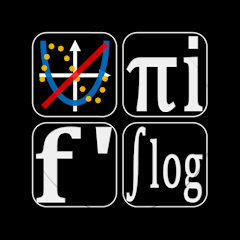# Graphing Calculator

10+Everyone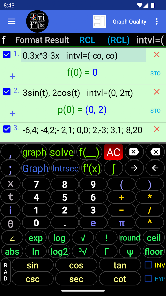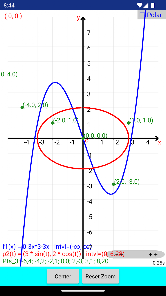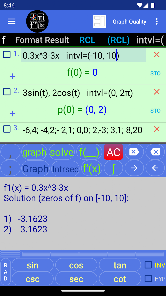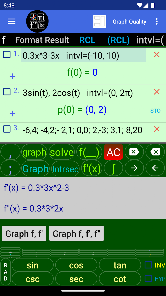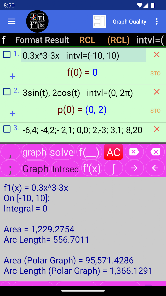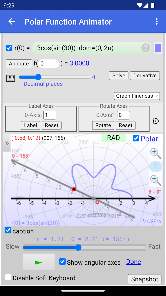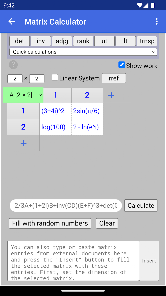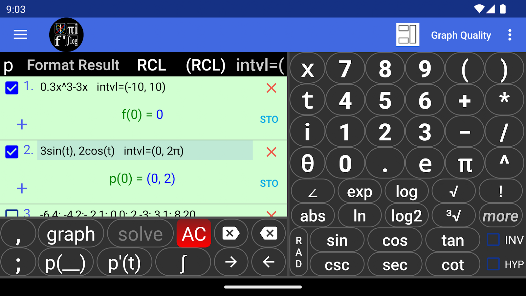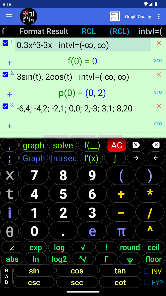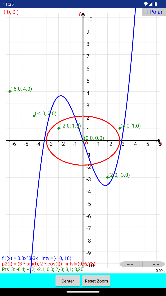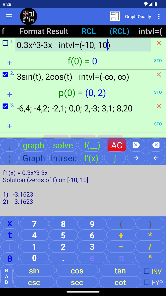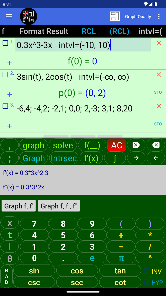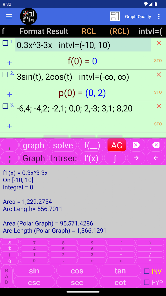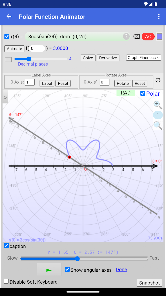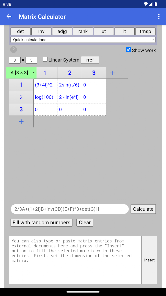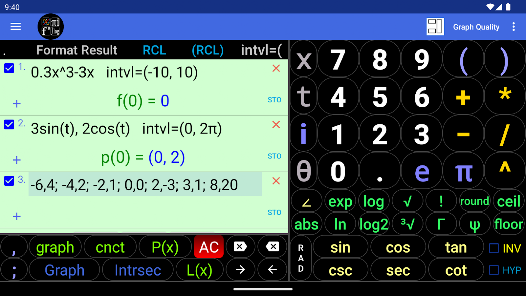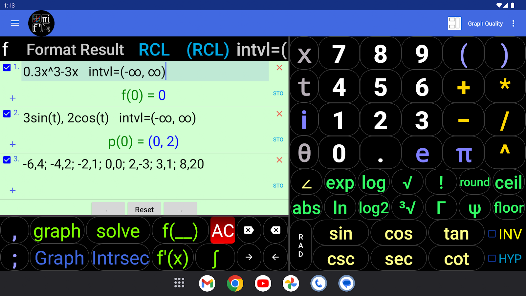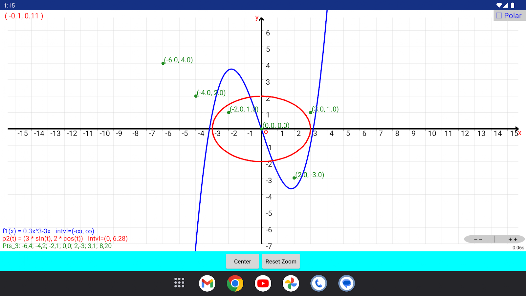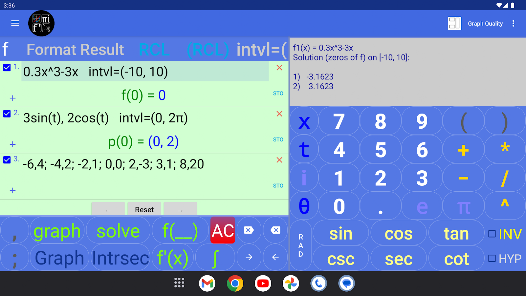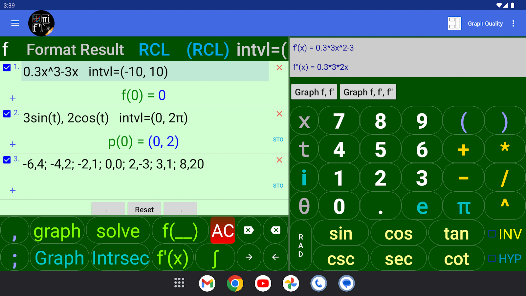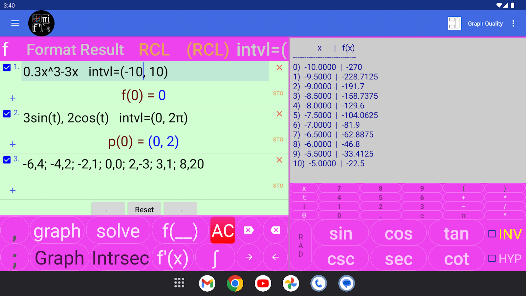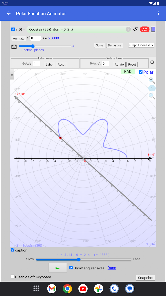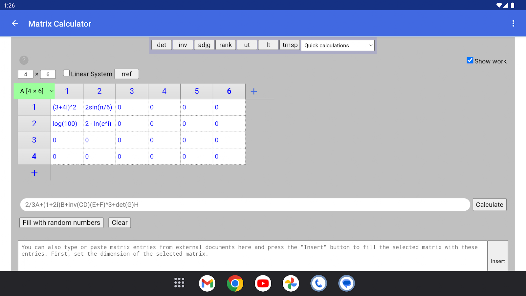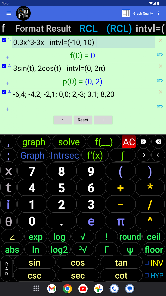The most powerful and the most easy-to-use free calculator app for 2D Cartesian and polar graphing on Android, and for doing matrix operations and solving systems of linear equations.

This graphing calculator features graphing capabilities offered by no other graphing calculators and its unique advanced user-friendly interface makes graphing and calculations as intuitive as possible.

Calculate and graph mathematical expressions containing
• basic algebraic operations
• linear and polynomial functions
• power functions, x^r and E(x)
• trigonometric functions and their inverses
• hyperbolic functions and their inverses
• exponential, e^x and exp(x), and logarithmic functions
• factorial
• the Gamma function, Γ
• the Psi functions, ψ
• absolute value and step functions: round, floor, ceil

This is the only graphing calculator that properly switches between the Cartesian and polar coordinate systems.

You can draw the Cartesian & polar graphs of
• functions
• parametric equations
• point sets

Also graph (needs Internet connection for the free version)
• equations
• explicitly defined functions
• conic sections: circles, ellipses, parabolas and hyperbolas in standard and general forms
• level curves

NOTE: to graph the expression in focus press the ‘graph’ button. To graph one or more selected expressions simultaneously, press the ‘Graph’ (capitalised) button.

This graphing calculator also implements graph animators that properly
• Animates polar graphs of functions by showing rotating angular axes
• Animates parametric graphs in the Cartesian coordinate system and also in polar coordinate system by showing rotating angular axes

With the help of the animators you can see how these type of graphs are progressively created and also Run, Pause and Resume the animations of polar and parametric graphs.

Calculate
• all the x-intercepts of the graph of a function on an interval with a single click by solving the equation f(x) = 0.
• points of intersection of the graphs of two functions.
• symbolic first and second order derivatives of functions and parametric equations and graph the derivatives.
• definite integrals
• area under (or enclosed by) the the Cartesian and polar graphs of functions (or parametric curves)
• arc length of Cartesian and polar graphs.

It is also the only graphing calculator capable of
• finding and graphing the polynomial of the least degree passing through a set of points.
• finding and graphing the best line (linear regression line or least squares line) that fits a set of points according to Gauss' Least Squares Criteria.

Other Features

• This scientific calculator is also capable of calculating complex numbers in standard, polar or any other forms.
• The calculator can display a table of values for functions and parametric equations even with complex expressions.
• The calculator can display the result in scientific, engineering and fixed point notations.
• Calculate relevant expressions in degrees or radians mode.

Matrices and Linear Systems (needs Internet connection for the free version)
This calculator also comes with a powerful matrix calculator capable of performing matrix algebra and solving systems of linear equations and shows work.
It is also the only matrix calculator capable of evaluating matrix expressions involving multiple matrices. It handles
• any m by n matrix
• complex matrices and linear systems with complex augmented matrices

Also calculate matrix
• determinant
• inverse
• rank
• reduced row echelon form
• triangular forms

The calculator also comes with an easy-to-use units converter (Time, Mass, Length, Velocity, and many more), and list of constants from various fields of sciences which can be used in your calculations.

Detailed built-in instruction is provided in the menu accompanying this graphing calculator.
Updated on
Mar 15, 2023

## Data safety

Safety starts with understanding how developers collect and share your data. Data privacy and security practices may vary based on your use, region, and age. The developer provided this information and may update it over time.No data shared with third parties